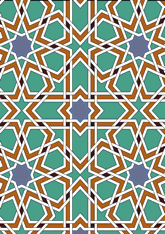# Algeria: gate of Bu-Medina at Tlemcendata210/CL54

## Geometry

• The symmetry group of the tiling is *442 (p4m).
• All the internal angles of the constituent polygons are a multiple of 22.5°.
• Contains one square.
• Contains four regular two-pointed star polygons with vertex angle of 45°.
• Contains two regular 8-pointed star polygons with vertex angle of 90°.
• There are 8 non-regular reflective tiles (including one kite) and two reflective pairs.
• The tiling satisfies the interlace condition and has no finite interlace and two infinite interlaces with straight cross-overs.
• The tiling is edge-to-edge.
• As drawn, contains about 372 polygons.

## References

Publications referenced:
1. Plate 54 (Bu-Medina, Tlemcen, Algeria. Gate) of J Collin. EÌtude pratique de la deÌcoration polygonale arabe, Paris, 1911. ISBN . [collin] {}

v53